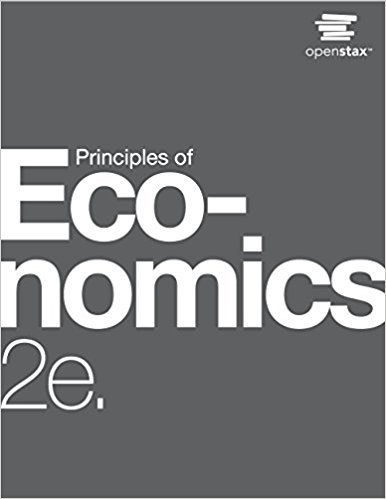×

×

# Solutions for Chapter D: The Expenditure-Output Model## Full solutions for Principles of Economics | 2nd Edition

ISBN: 9781947172364Solutions for Chapter D: The Expenditure-Output Model

Solutions for Chapter D
4 5 0 243 Reviews
12
4
##### ISBN: 9781947172364

This textbook survival guide was created for the textbook: Principles of Economics, edition: 2. This expansive textbook survival guide covers the following chapters and their solutions. Principles of Economics was written by and is associated to the ISBN: 9781947172364. Since 27 problems in chapter D: The Expenditure-Output Model have been answered, more than 16256 students have viewed full step-by-step solutions from this chapter. Chapter D: The Expenditure-Output Model includes 27 full step-by-step solutions.

Key Business Terms and definitions covered in this textbook
• agent

a person who is performing an act for another person, called the principal

• budget constraint

the limit on the consumption bundles that a consumer can afford

• budget surplus

an excess of tax revenue over government spending

• Coase theorem

the proposition that if private parties can bargain without cost over the allocation of resources, they can solve the problem of externalities on their own

• complements

two goods for which an increase in the price of one leads to a decrease in the demand for the other

• consumer surplus

the amount a buyer is willing to pay for a good minus the amount the buyer actually pays for it

• corrective tax

a tax designed to induce private decision makers to take account of the social costs that arise from a negative externality

• demand curve

a graph of the relationship between the price of a good and the quantity demanded

• economic profit

total revenue minus total cost, including both explicit and implicit costs

• equilibrium price

the price that balances quantity supplied and quantity demanded

• marginal cost

the increase in total cost that arises from an extra unit of production

• Nash equilibrium

a situation in which economic actors interacting with one another each choose their best strategy given the strategies that all the other actors have chosen

• perfect complements

two goods with right-angle indifference curves

• political economy

the study of government using the analytic methods of economics

• property rights

the ability of an individual to own and exercise control over scarce resources

a theory of exchange rates whereby a unit of any given currency should be able to buy the same quantity of goods in all countries

• risk aversion

a dislike of uncertainty

• supply curve

a graph of the relationship between the price of a good and the quantity supplied